The Physics of a Golf Putt

Introduction

In many respects, golf is the perfect sport to analyze using physics. The interactions between a club and a golfer, the club and the ball, and the ball and the surface are all consistent and repeatable, and involve only a few bodies. Despite this convenience, physics is rarely applied directly to the game itself. Until very recently, almost all developments in golfing technology were made using trial and error, rather than through a rigorous scientific approach . Furthermore, the application of physics during the game itself is almost unheard of. It is rare for one to see a golfer take out pen and paper on the tee box in order to predict the trajectory of his upcoming drive. (Indeed, this author would venture to predict that there is an inverse relationship between the ability of the player to model his own drive and the likelihood that their drive will be a good one.) Rather than applying physics during the game itself, it is instead more reasonable to apply physics in order to determine the statistical likelihood of certain outcomes, or to determine the appropriate action for the average player. In this paper, the physics of a level putt will be examined. By modeling the physics of a golf ball rolling on a green, and the interaction between the ball and the cup, the conditions required for a successful putt will be predicted.

A Golf Ball Rolling on a Level Green

An American golf ball is a sphere of radius 0.0427m , with dimples in it in order to improve loft. A putt in golf consists of striking the golf ball with a flat-faced club known as a putter. Most putters have a slightly lofted face, which result in the ball becoming slightly airborne when struck, and bouncing several times on the green before settling into a state of pure rolling . Some putters have no loft, in which case the ball will initially be in a combined state of sliding and rolling before settling into a perfect roll. Analysis of both methods of putting indicate that the ball will be in a pure state of rolling after approximately 20% of its total path length has been traveled . In order to simplify the problem of modeling a putt, the ball will be treated as a perfect sphere, and will be considered to be in a perfect state of rolling immediately after being struck. This will likely only have second order effects on the accuracy of the predictions .

A sphere rolling on a surface is slowed and eventually brought to a stop by rolling friction. Rolling friction appears as a torque opposed to the sphere's motion of the form T= ρ n, where ρ is the coefficient of rolling friction and n is the normal force exerted by the surface on the sphere . This frictional torque can be explained by assuming that the sphere and the plane are both slightly deformed by their contact with each other, so that there is a small depression in both. The reaction force of the plane is therefore applied to a point slightly in front of the ideal point of contact. ρ then gives the position of the equivalent single contact point on the golf ball, which is the perpendicular distance between the normal component of the contact force and the center of mass of the golf ball . The equations of motion for a golf ball of mass m and radius R are given by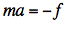(1)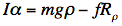(2)

where Rρ is the perpendicular distance between the tangential component of the contact force f and the center of mass of the golf ball, and is given by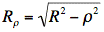(3)

Typical values of ρ are in the range 10-2 to 10-4 cm, which means that ρ < < R , and so the approximation Rρ = R can be made. In order for the ball to be rolling without slipping, we must have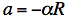(4)

Solving eqs. (1), (2) and (4) for the acceleration a of the golf ball gives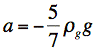(5)

where ρg=ρ/R.

In golf, golfers often refer to the speed of a green, with a fast green meaning a green on which the ball travels a long distance before coming to a rest, and a slow green meaing a green on which the ball comes to rest after only a short distance. The speed of a green is directly related the deceleration of the golf ball, and is therefore a measure of the value of ρg. A device called a stimpmeter, which is basically an inclined plane with a grove down its center, typically measures the speed of a green. The stimpmeter always releases the ball at a constant speed as it leaves the inclined plane. This speed has been measured to be 1.83 m/s . For what can be considered a very fast green a ball will roll approximately 3.66 m after leaving the stimpmeter, while for a very slow green a ball will only roll approximately 1.22 m . Using the extreme rolling distances and the speed of the golf ball as it leaves the stimpmeter, the ranges of ρg can be found to be 0.065 < ρg < 0.196, with an average value of 0.131.

Interaction Between the Ball and the Hole

Once a ball has been directed towards a hole at a specific velocity, the question becomes whether the hole will capture the ball when the ball interacts with it. The conditions for the capture of a golf ball by a hole of radius RH have been considered extensively by B.W. Holmes . The simplest condition of capture for a ball traveling directly at the center of a hole is that after the ball leaves the front rim it must fall a distance greater than its radius R before impacting the back rim, or else it will bounce up and out. For a ball traveling at velocity vf when it reaches the hole, the time the ball will be in free fall before it impacts the back rim is given by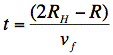(6)

The condition for capture by free fall is therefore given by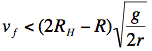(7)

Plugging in the standard USGA hole radius of 0.0427 m and ball radius of 0.02135 m gives the capture condition vf < 1.31 m/s. Using computer simulation, Holmes was able to account for additional methods of ball capture, such as the ball rolling around the lip, using computer simulation. In the more general case of off center impacts, the condition for the capture of the ball by free fall is shown by Holmes to be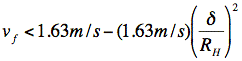(8)

where δ is the impact parameter.

Conditions for a Successful Putt

Using eqs. (5) and (8) the conditions for a successful putt from a distance d away from the hole can now be determined. Using eq. (5), a ball putted with initial velocity vo will have a final velocity when it reaches the hole given by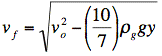(9)

For a putt that is directly on target the impact parameter is zero, and eq. (8) can used to determine the required final velocity for a successful capture. Plugging eq. (9) into eq. (8) gives a required launch speed vo of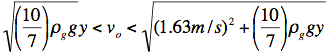(10)

The range of possible launch angles for a hole a distance that lead to an interaction between the ball and the hole which could lead to a successful capture are given by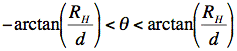(11)

Taken together, eqs. (10) and (11) help explain why it becomes more and more difficult to make a putt as the distance increases. Not only must the green be read properly, but even for a perfectly level green the range of successful launch speeds vo and successful launch angles θ become smaller as d increases. In the case where the chance of making the putt is small, the imprecision of the golfer's control over the launch angle and launch speed will be much larger than the range of launch conditions required for a successful putt. In these cases, the probability of the player making a putt will be proportional to the area of the required launch conditions, given in launch-speed launch-angle space. Combining eqs. (8) and (11), A. Penner was able to determine the theoretical relative areas for level putts ranging from 6 to 30 ft in length, and compare the theoretical probabilities with the range of success of professional golfers in making putts at these same distances . He found that the theoretical success rates are in excellent agreement with the professional success rates.

Conclusion:

By modeling a golf ball as a perfect sphere, the equations of motion for a golf ball rolling on a level surface were found. These equations were used in conjunction with Holmes' criteria for ball capture  to determine the initial required velocities and angles for a successful putt from a distance d away from the hole. The results of this analysis demonstrate why a putt becomes more difficult as the distance to the hole is increased, even when putting on a level green. A comparison made by A. Penner  between the theoretical results and actual professional success rates shows good agreement between theory and reality.

© 2007 Shimon Kolkowitz. The author grants permission to copy, distribute and display this work in unaltered form, with attribution to the author, for noncommercial purposes only. All other rights, including commercial rights, are reserved to the author.

References

 A. R. Penner, "The Physics of Golf," Rep. Prog. Phys. 66, 131 (2003).

 B. W. Holmes, "Putting: How a Golf Ball and Hole Interact," Am. J. Phys. 59, 129 (1991).

 A. R. Penner, "The Physics of Putting," Can. J. Phys. 80, 83 (2002).

 A. Domenech, T. Domenech and J. Cebrian. "Introduction to the Study of Rolling Friction," Am. J. Phys. 55, 231 (1987).